# Python and the Financial Industry¶

A Subjective and Biased Overview

Dr. Yves J. Hilpisch | The Python Quants GmbH

Pycon Ireland, 11. October 2014

## The Python Language¶

Black-Scholes-Merton (1973) SDE of geometric Brownian motion.

$$dS_t = rS_tdt + \sigma S_t dZ_t$$

Monte Carlo simulation: draw $I$ standard normally distributed random number $z_t^i$ and apply them to the following by Euler disctretization scheme to simulate $I$ end values of the GBM:

$$S_{T} = S_0 \exp \left(\left( r - \frac{1}{2} \sigma^2\right) T + \sigma \sqrt{T} z_T \right)$$

Latex description of Euler discretization.

S_T = S_0 \exp (( r - 0.5 \sigma^2 ) T + \sigma \sqrt{T} z_T)

Python implementation of algorithm.

In :
from pylab import *
S_0 = 100.; r = 0.01; T = 0.5; sigma = 0.2
z_T = standard_normal(10000)

In :
S_T = S_0 * exp((r - 0.5 * sigma ** 2) * T + sigma * sqrt(T) * z_T)


Again, Latex for comparison:

S_T = S_0 \exp (( r - 0.5 \sigma^2 ) T + \sigma \sqrt{T} z_T)

Interactive visualization of simulation results.

In :
%matplotlib inline
pyfig = figure()
hist(S_T, bins=40);
grid()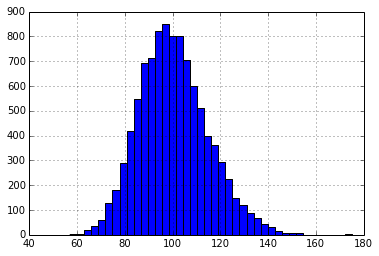## The Python Ecosystem¶

• IPython (Notebook)
• NumPy (fast, vectorized array operations)
• SciPy (collection of scientific classes/functions)
• pandas (times series and tabular data)
• PyTables (hardware-bound IO operations)
• scikit-learn (machine learning algorithms)
• statsmodels (statistical classes/functions)
• xlwings (Python-Excel integration)

## Financial Libraries¶

By others:

• zipline (backtesting of trading algos)
• matplotlib.finance (financial plots)
• Python wrappers (QuantLib)

By us:

• DEXISION – GUI-based financial engineering
• DX Analytics – global valuation of multi-risk derivatives and portfolios

### Example: DX Analytics¶

DX Analytics is a Python library for advanced financial and derivatives analytics written by The Python Quants. It is particularly suited to model multi-risk derivatives and to do a consistent valuation of portfolios of complex derivatives. It mainly uses Monte Carlo simulation since it is the only numerical method capable of valuing and risk managing complex, multi-risk derivatives books.

An example with an European maximum call option on two underlyings.

In :
%%time
import dx
%run dx_example.py
# sets up market environments
# and defines derivative instrument
# calculates a number of numerical results

CPU times: user 4.9 s, sys: 335 ms, total: 5.24 s
Wall time: 5.26 s


In :
max_call.payoff_func
# payoff of a maximum call option
# on two underlyings (European exercise)

Out:
"np.maximum(np.maximum(maturity_value['gbm'], maturity_value['jd']) - 34., 0)"

In :
max_call.vega('jd')
# numerical Vega with respect
# to one risk factor

Out:
4.194600000000115


A Vega surface for one risk factor with respect to the initial values of both risk factors.

In :
dx.plot_greeks_3d([a_1, a_2, vega_gbm], ['gbm', 'jd', 'vega gbm'])
# Vega surface plot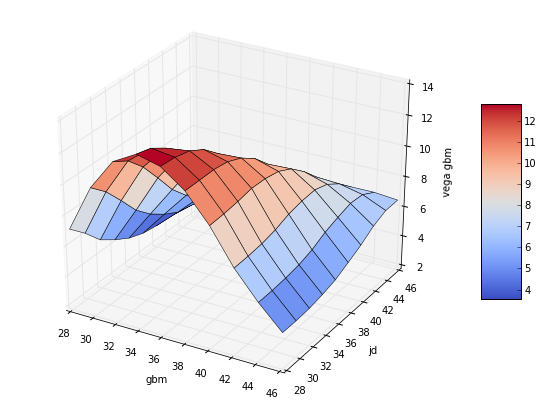## APIs – Interfacing with Others

• Thomson Reuters (wrapper for unified API in the making)
• Front Arena (scripting with Python)
• Murex (scripting payoffs with Python)
• ...

### Example: Murex¶

From http://www.risk.net:

"Murex provides a complete cross-asset and front-to-back offering for structured products, combining out-of-the box complex payoffs and models with structuring tools, and model and products catalogue extensors.

Key features include:

• A wide native catalogue of exotic products and best-of-breed models, all compliant with a grid.
• A generic Monte Carlo, fully compliant with graphics processing units, providing impressive performance speed-ups.
• A structured trade builder to create any on-the-fly packages, persistent contracts and structured over-the-counter trades or securities – for example, warrants and bonds.
• A payoff language to describe any complex exotic with an interpreted language (Python) in an unbeatable time to market, and for both revaluation and front-to-back integration."

## Integration – No "Either Or" with Python

• C/C++ (natively)
• Julia (IPython)
• JavaScript (IPython)
• R (IPython/rpy2)
• Matlab (NumPy)
• ...

### Example: Statistics with R¶

We analyze the statistical correlation between the EURO STOXX 50 stock index and the VSTOXX volatility index.

First the EURO STOXX 50 data.

In :
import pandas as pd
cols = ['Date', 'SX5P', 'SX5E', 'SXXP', 'SXXE',
'SXXF', 'SXXA', 'DK5F', 'DKXF', 'DEL']
try:
index_col=0,  # index column (dates)
parse_dates=True,  # parse these dates
dayfirst=True,  # format of dates
skiprows=4,  # ignore these rows
sep=';',  # data separator
names=cols)  # use these column names

# deleting the helper column
del es['DEL']
except:
# read stored data if there is no Internet connection
es = pd.HDFStore('data/SX5E.h5', 'r')['SX5E']


Second, the VSTOXX data.

In :
vs_url = 'http://www.stoxx.com/download/historical_values/h_vstoxx.txt'

try:
index_col=0,  # index column (dates)
parse_dates=True,  # parse date information
dayfirst=True, # day before month
except:
# read stored data if there is no Internet connection
vs = pd.HDFStore('data/V2TX.h5', 'r')['V2TX']


Generating log returns with Python and pandas.

In :
import numpy as np
# log returns for the major indices' time series data
datv = pd.DataFrame({'SX5E' : es['SX5E'], 'V2TX': vs['V2TX']}).dropna()
rets = np.log(datv / datv.shift(1)).dropna()
ES = rets['SX5E'].values
VS = rets['V2TX'].values


Bridging to R from within IPython Notebook and pushing Python data to the R run-time.

In :
%load_ext rpy2.ipython

The rpy2.ipython extension is already loaded. To reload it, use:


In :
%Rpush ES VS


Plotting with R in IPython Notebook.

In :
%R plot(ES, VS, pch=19, col='blue'); grid(); title("Log returns ES50 & VSTOXX")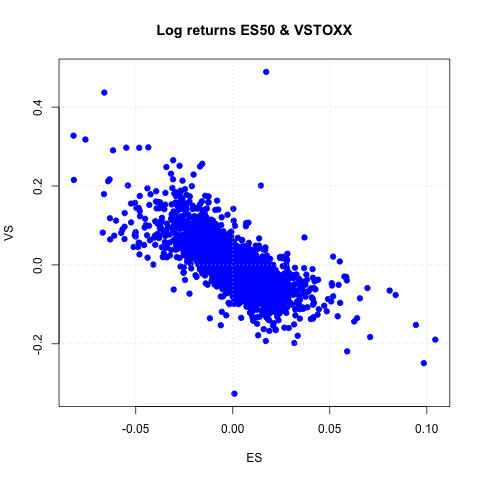Linear regression with R.

In :
%R c = coef(lm(VS~ES))

Out:
<FloatVector - Python:0x10b247680 / R:0x10e9135c8>
[-0.000057, -2.756117]

In :
%R print(summary(lm(VS~ES)))


Call:
lm(formula = VS ~ ES)

Residuals:
Min       1Q   Median       3Q      Max
-0.32413 -0.02192 -0.00215  0.02018  0.53679

Coefficients:
Estimate Std. Error t value Pr(>|t|)
(Intercept) -5.661e-05  6.160e-04  -0.092    0.927
ES          -2.756e+00  4.073e-02 -67.661   <2e-16 ***
---
Signif. codes:  0 ‘***’ 0.001 ‘**’ 0.01 ‘*’ 0.05 ‘.’ 0.1 ‘ ’ 1

Residual standard error: 0.03905 on 4016 degrees of freedom
Multiple R-squared:  0.5327,	Adjusted R-squared:  0.5326
F-statistic:  4578 on 1 and 4016 DF,  p-value: < 2.2e-16



Regression line visualized.

In :
%R plot(ES, VS, pch=19, col='blue'); grid(); abline(c, col='red', lwd=5)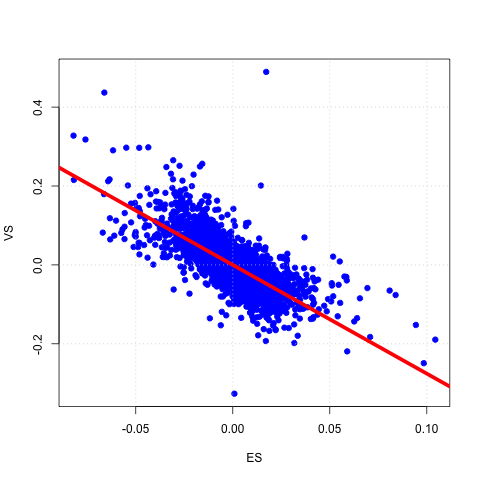Pulling data from R to Python and using it.

In :
%Rpull c

In :
plt.figure(figsize=(9, 6))
plt.plot(ES, VS, 'b.')
plt.plot(ES, c + c * ES, 'r', lw=3)
plt.grid(); plt.xlabel('ES'); plt.ylabel('VS')

Out:
<matplotlib.text.Text at 0x105ee14d0>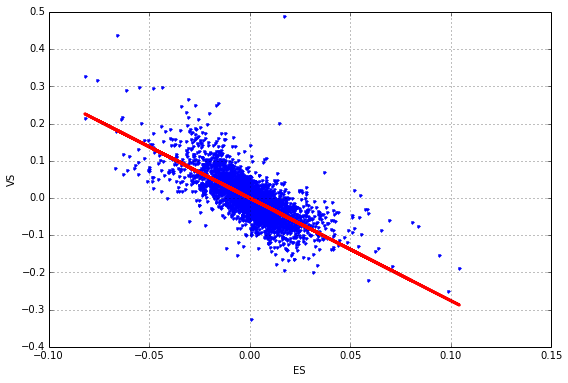If you want to have it nicer, interactive and embeddable anywhere – use plot.ly

In :
import plotly.plotly as ply
ply.sign_in('yves', 'token')


Let us generate a plot with a bit fewer data points.

In :
pyfig = plt.figure(figsize=(9, 6)); n = 100
plt.plot(ES[:n], VS[:n], 'b.')
plt.plot(ES[:n], c + c * ES[:n], 'r', lw=3)
plt.grid(); plt.xlabel('ES'); plt.ylabel('VS')

Out:
<matplotlib.text.Text at 0x110d83f10>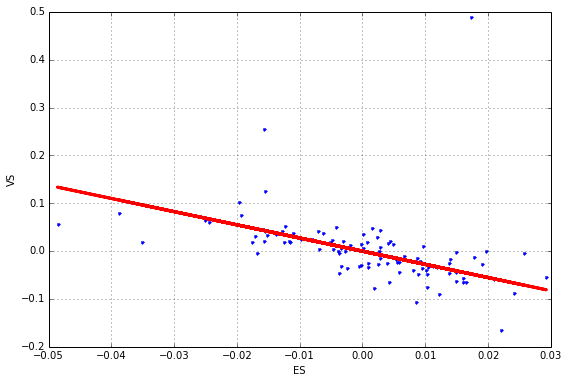Only single line of code needed to convert matplotlib plot into interactive D3 plot.

In :
ply.iplot_mpl(pyfig)  # convert mpl plot into interactive D3

Out:

## Performance – Numerical Algorithms

Finance algorithms are loop-heavy; Python loops are slow; Python is too slow for finance.

In :
def counting_py(N):
s = 0
for i in xrange(N):
for j in xrange(N):
s += int(cos(log(1)))
return s

In :
N = 2000
%time counting_py(N)
# memory efficient but slow

CPU times: user 12.9 s, sys: 222 ms, total: 13.1 s
Wall time: 13.1 s


Out:
4000000


First approach: vectorization with NumPy.

In :
%%time
arr = ones((N, N))
print int(sum(cos(log(arr))))

4000000
CPU times: user 75.4 ms, sys: 44.8 ms, total: 120 ms
Wall time: 119 ms


In :
arr.nbytes # much faster but NOT memory efficient

Out:
32000000


Second approach: dynamic compiling with Numba.

In :
import numba
counting_nb = numba.jit(counting_py)

In :
%time counting_nb(N)
# some overhead the first time

CPU times: user 140 ms, sys: 12.8 ms, total: 153 ms
Wall time: 144 ms


Out:
4000000

In :
%timeit counting_nb(N)
# even faster AND memory efficient

10 loops, best of 3: 59.2 ms per loop



## Performance – Hardware-bound IO

Hardware-bound IO operations are standard for Python.

In :
%time one_gb = standard_normal((12500, 10000))
one_gb.nbytes
# a giga byte worth of data

CPU times: user 5.27 s, sys: 373 ms, total: 5.65 s
Wall time: 5.65 s


Out:
1000000000

In :
%time save('one_gb', one_gb)

CPU times: user 53.5 ms, sys: 1.72 s, total: 1.77 s
Wall time: 2.23 s


In :
!ls -n one_gb*

-rw-r--r--  1 501  20  1000000080 10 Okt 19:11 one_gb.npy



## Python Quant Platform¶

Integrating it all and adding collaboration and scalability (http://quant-platform.com).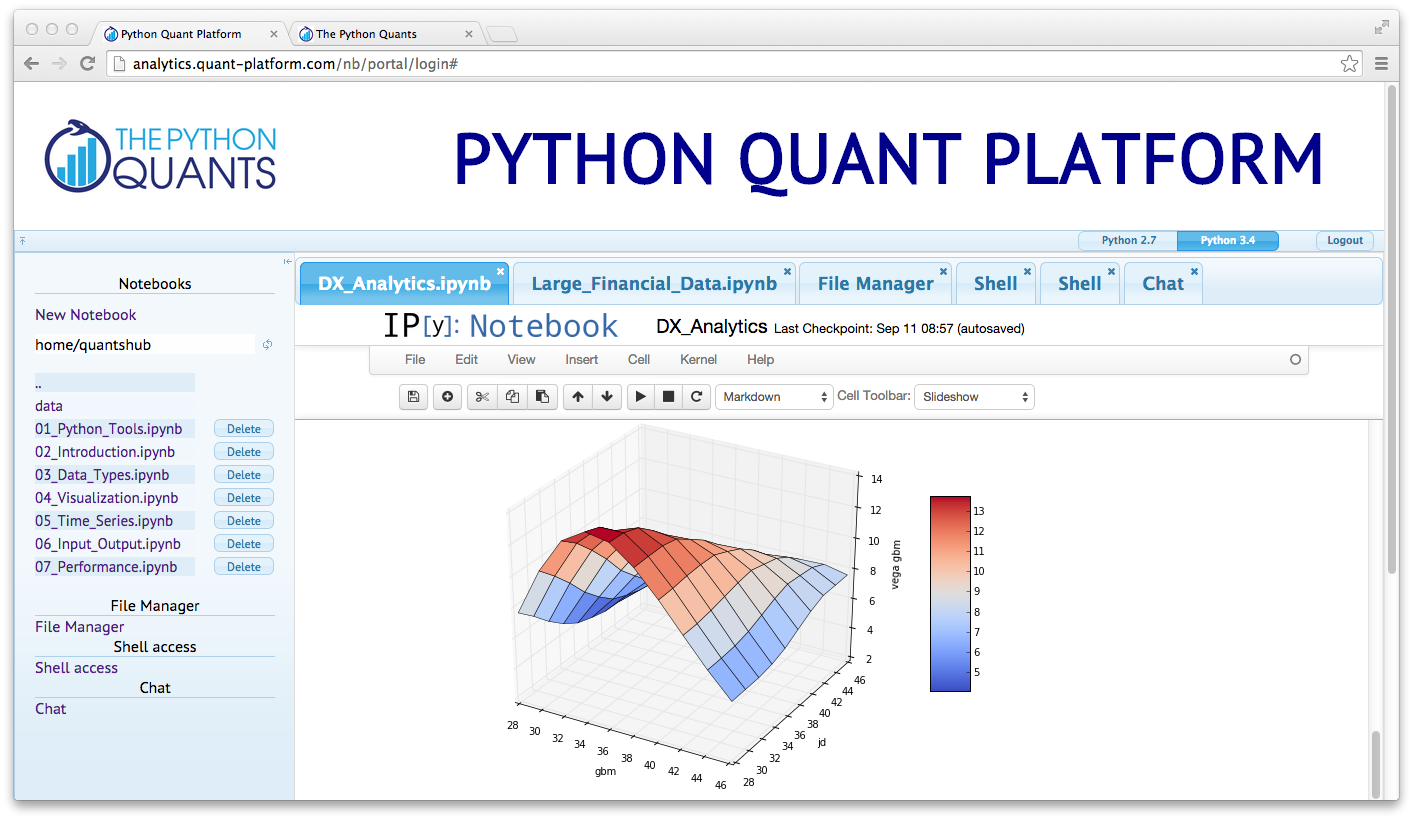At the moment, the Python Quant Platform comprises the following components and features:

• IPython Notebook: interactive data and financial analytics in the browser with full Python integration and much more (cf. IPython home page).
• IPython Shell, Python Shell, System Shell: all you typically do on the (local or remote) system shell (Vim, Git, file operations, etc.)
• Anaconda Python Distribution: complete Python stack for financial, scientific and data analytics workflows/applications (cf. Anaconda page); you can easily switch between Python 2.7 and 3.4.
• R Stack: for statistical analyses, integrated via rpy2 and IPython Notebook
• DX Analytics: our library for advanced financial and derivatives analytics with Python based on Monte Carlo simulation.
• File Manager: a GUI-based File Manager to upload, download, copy, remove, rename files on the platform.
• Chat/Forum: there is a simple chat/forum application available via which you can share thoughts, documents and more.
• Collaboration: the platform features user/group administration as well as file sharing via public folders.
• Linux Server: the platform is powered by Linux servers to which you have full shell access.
• Deployment: the platform is easily scalable since it is cloud-based and can also be easily deployed on your own servers (via Docker containers).

Working on the browser-based shell and using, for instance, IPython.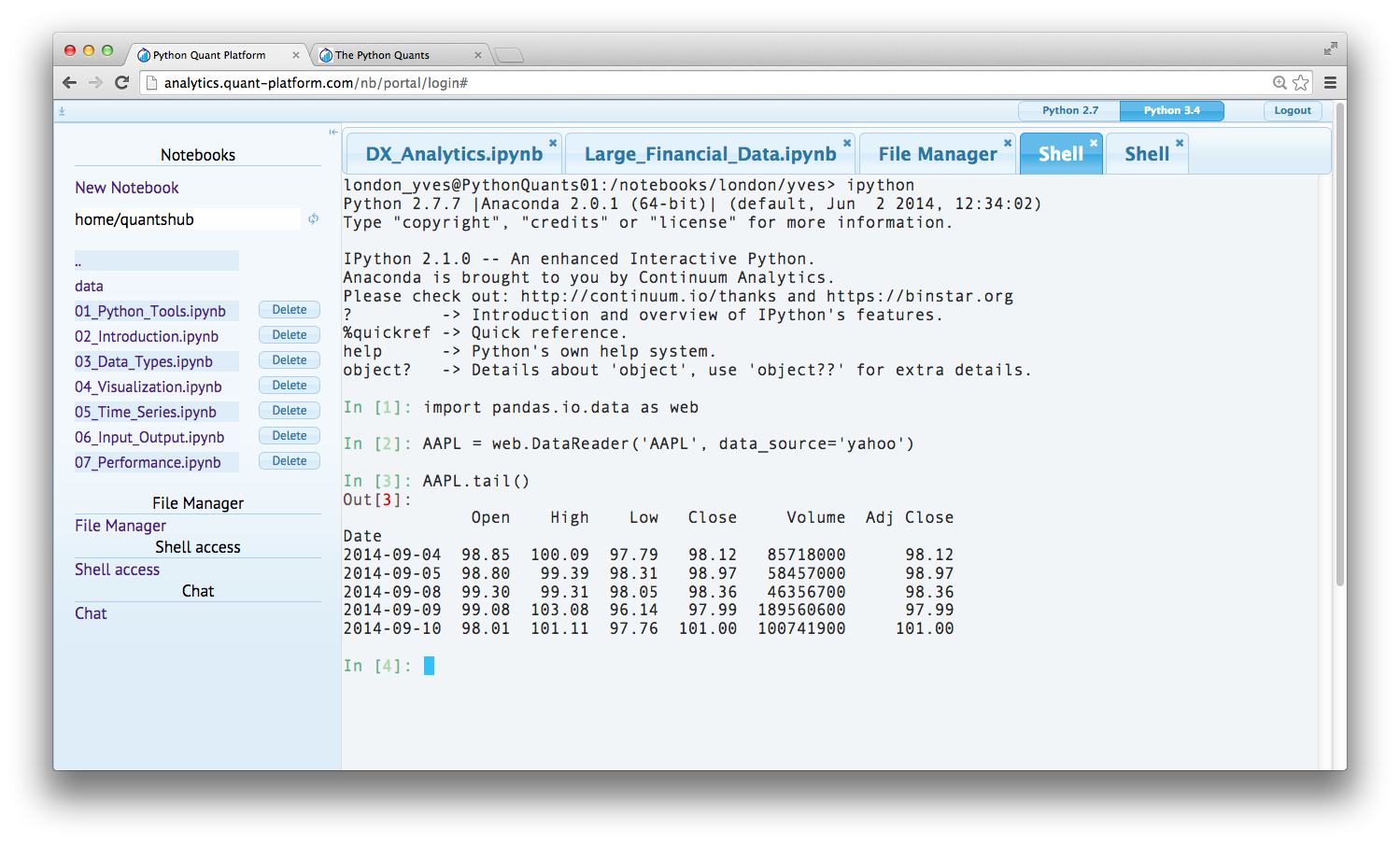Or doing code editing with Vim, working with Git, etc.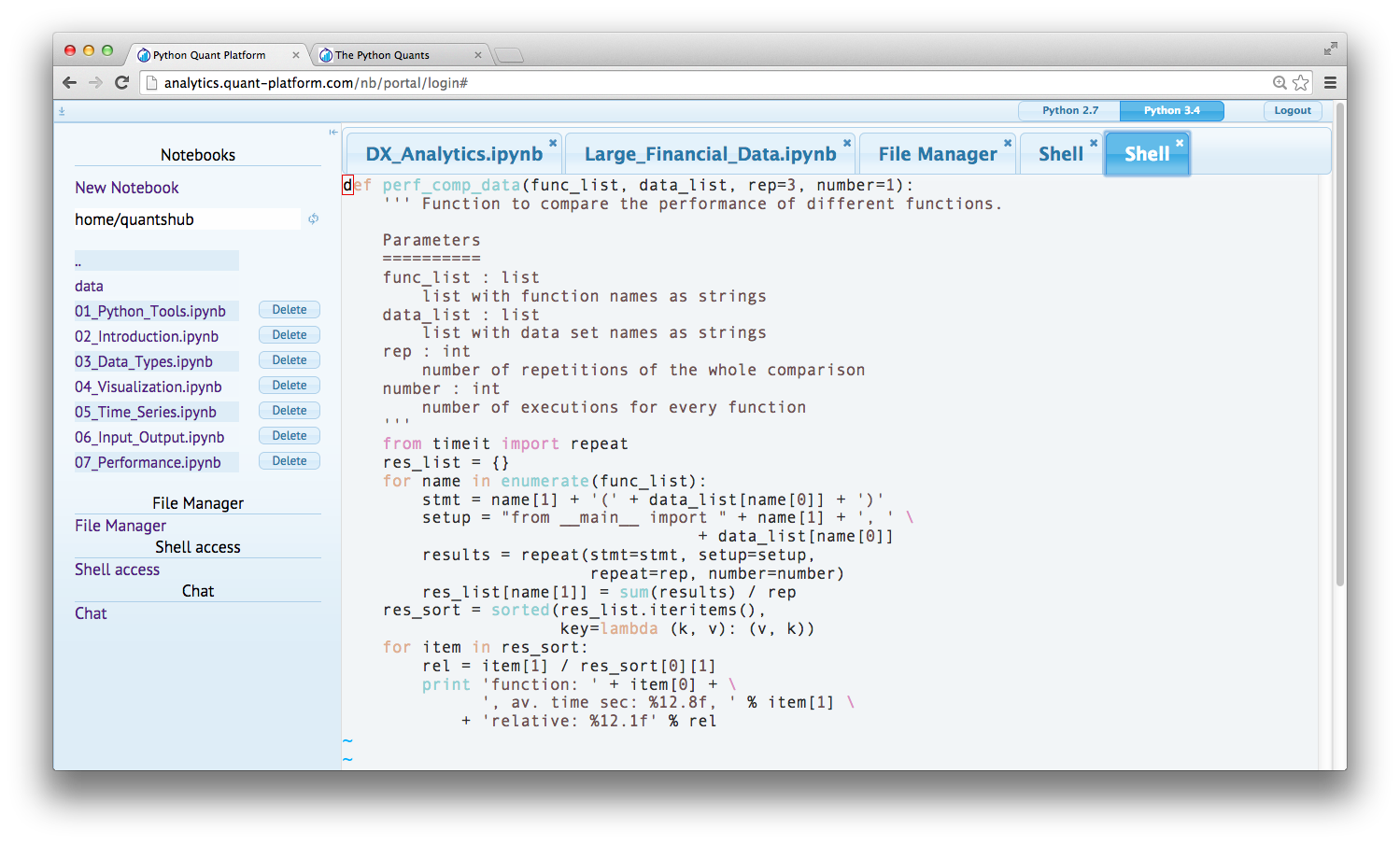Or checking in on resource usage with htop.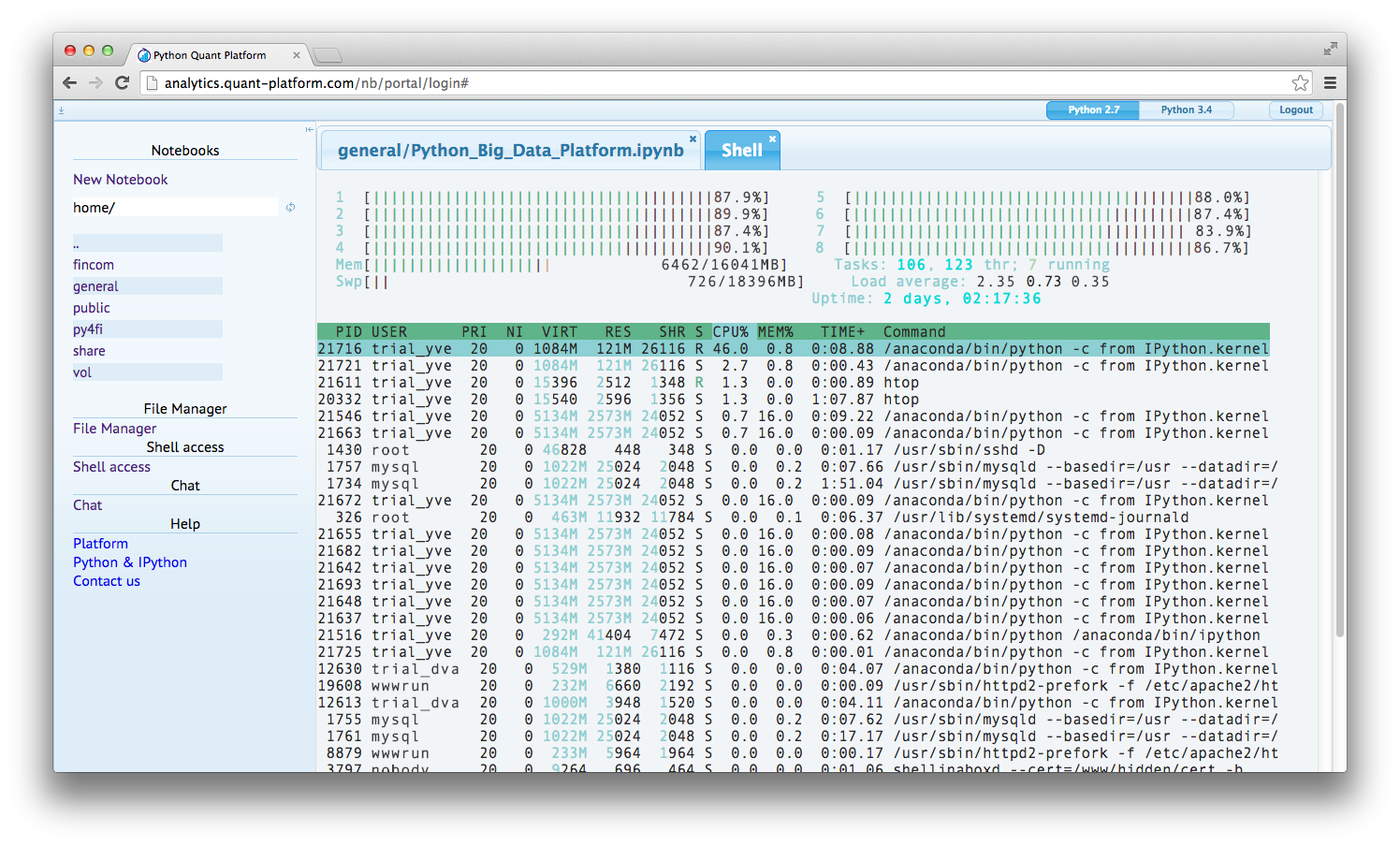In :
from IPython.display import HTML
HTML('<iframe src=http://analytics.quant-platform.com/trial/yves/ \
width=100% height=550></iframe>')

Out:

## The Large Banks¶

• JPMorgan Chase (Athena platform)
• Bank of America Merrill Lynch (Quartz platform)
• Lloyds Bank
• BNP Paribas
• Nomura
• ...

### Example: JPMorgan¶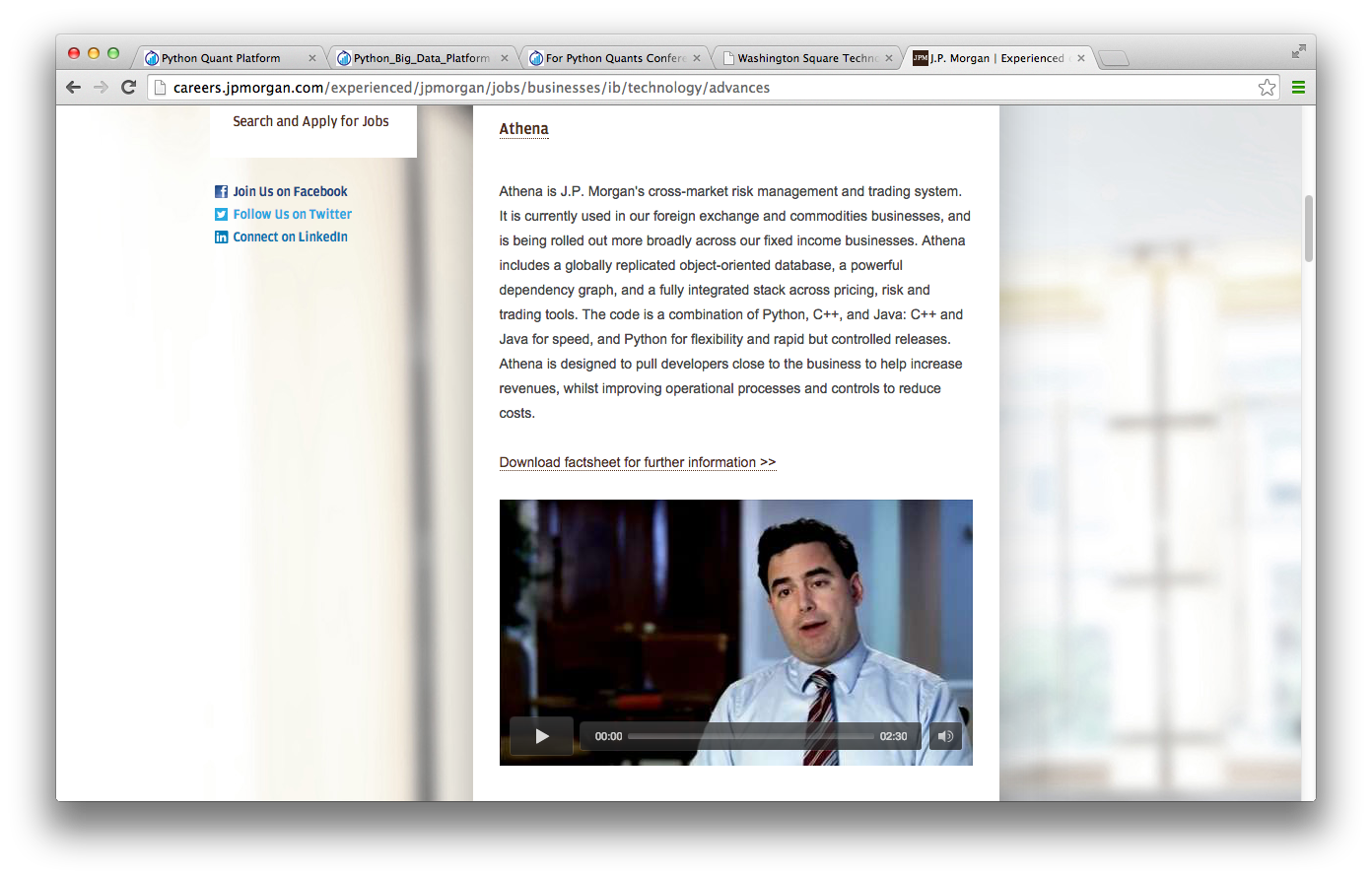### Example: Bank of America Merrill Lynch¶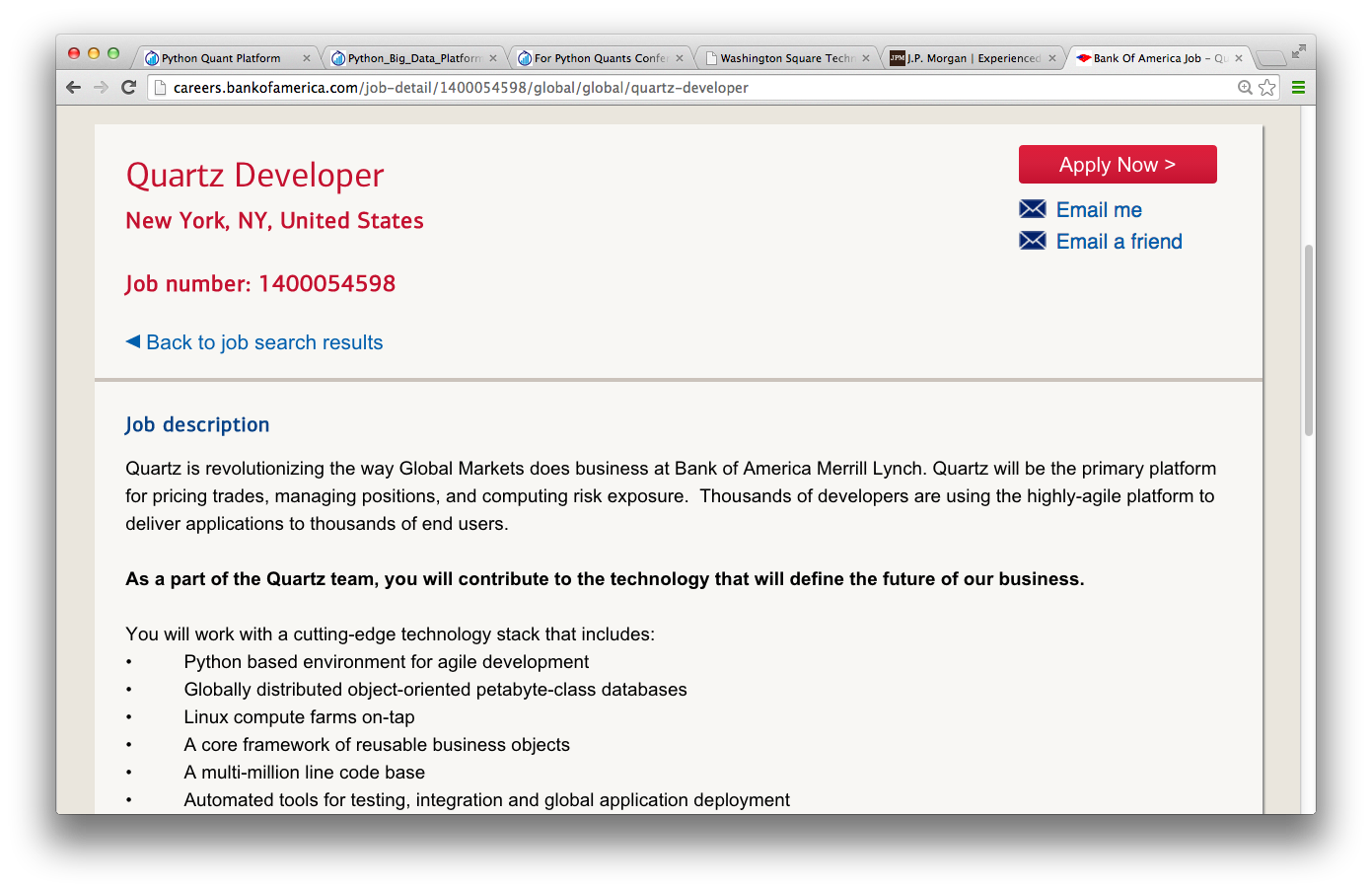## The Hedge Funds¶

• AQR Capital Management (origin of pandas)
• Two Sigma Investments (large scale data analytics)
• ARC Investments (full-fledged Python for vol trading)
• ...

### Example: Python Jobs in Greenwich (CT)¶

392 Python Jobs in Greenwich, CT – obviously a famous hedge fund place.## Innovators in the Space¶

• Quantopian (algo trading & backtesting)
• Washington Square Technologies (trade & risk platform)
• Deutsche Boerse/Eurex (VSTOXX and Variance Advanced Services)
• ...

### Example: Quantopian¶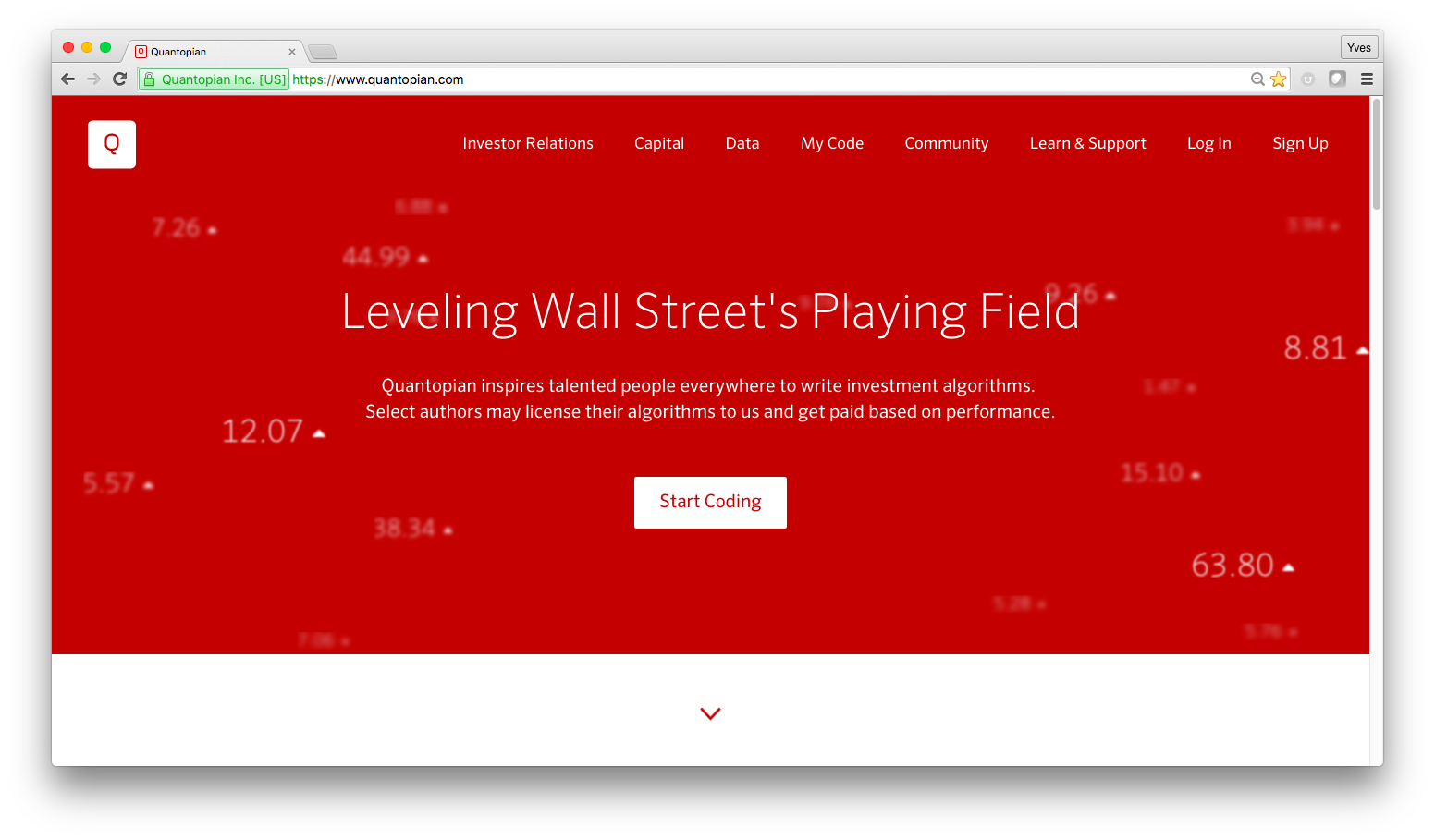### Example: Washington Square Technologies¶

Trading and Risk Management powerd by Python.### Example: Eurex Advanced Services (VSTOXX & Variance Futures)¶

Python-based tutorials by Eurex (http://www.eurexchange.com/vstoxx/).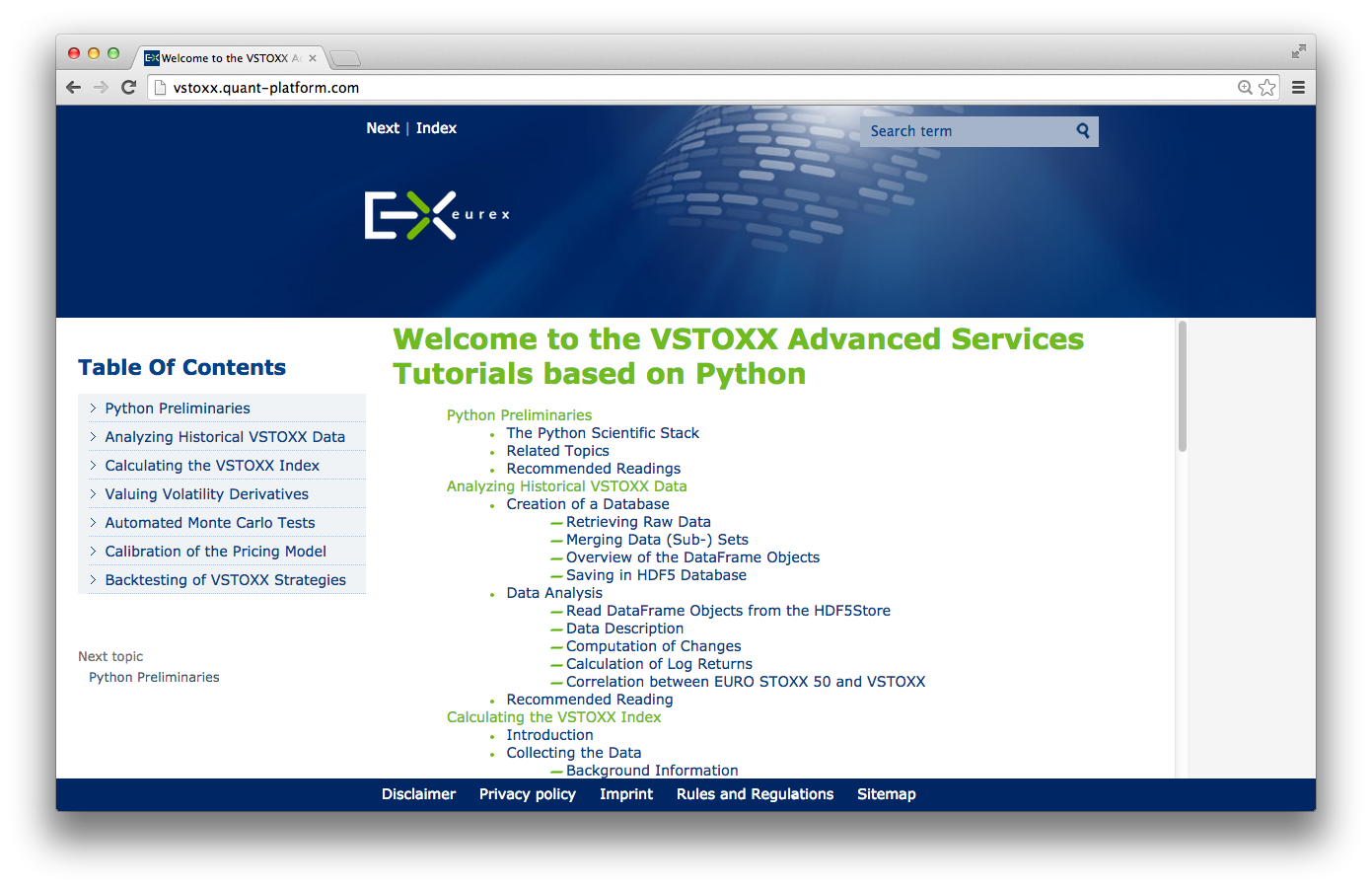## Books¶

By others:

• Python for Financial Modelling @ Wiley Finance (2009)
• Python for Finance @ Packt Publishing (2014)

By myself:

• Python for Finance – Analyze Big Financial Data @ O'Reilly (2014)
• Derivatives Analytics with Python @ Wiley Finance (2015)

Available as ebook and from December 2015 as print version (currently 50% discount – see my Twitter account @dyjh).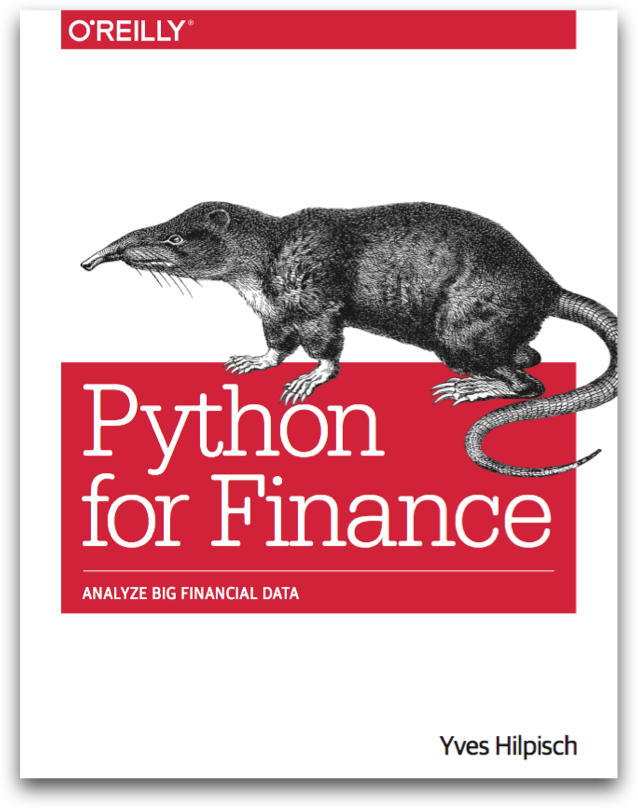Forthcoming 2015 at Wiley Finance ...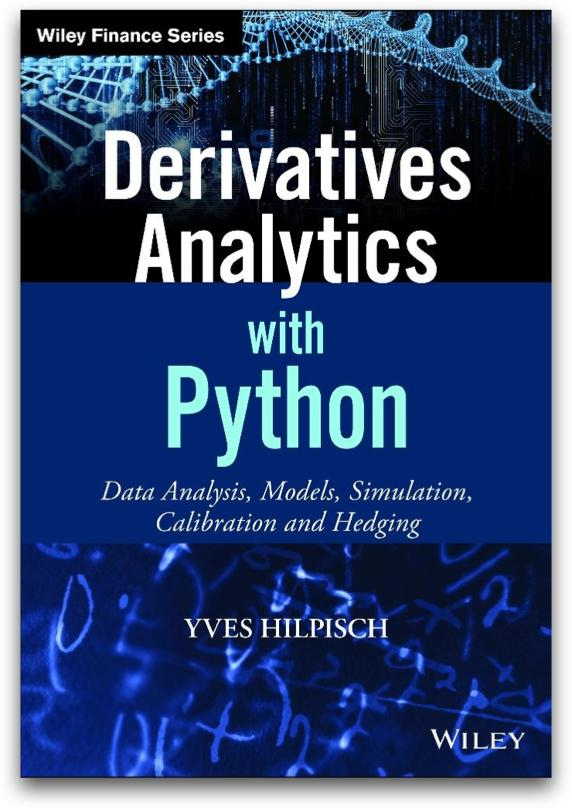## Financial Research¶

"The appendices present an implementation in Python of our experiments." (p. 3)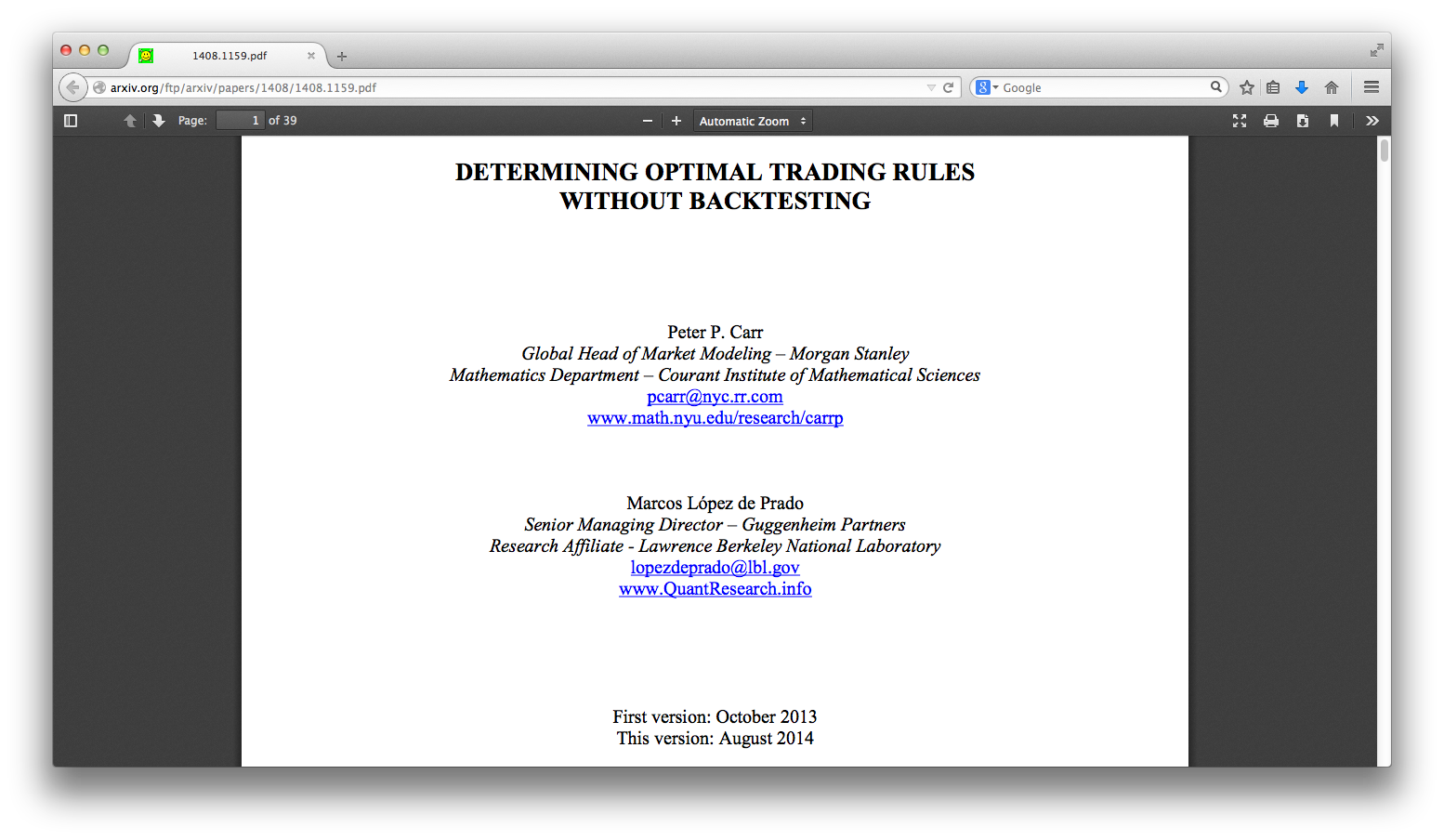## Education¶

• Master of Financial Engineering @ Baruch College CUNY
• Master of Data Science @ City University of New York
• Numerical Option Pricing with Python @ Saarland University
• ...

"Knowledge and Skills: Our graduates have working experience with C++, VBA, Python, R, and Matlab for financial applications. They share an exceptionally strong work ethic and possess excellent interpersonal, teamwork, and communication skills."

## Training¶

By others:

• Python for Finance @ Continuum Analytics
• Python for Finance @ Enthought

By myself:

• Python for Quant Finance @ Python for Quant Finance Meetup Group
• Python for Finance @ http://quantshub.com
• NumPy & pandas for Finance @ CQF Institute/Fitch Learnings
• ...
In :
HTML('<iframe src=http://quantshub.com/content/python-finance-yves-j-hilpisch \
width=100% height=550></iframe>')

Out:

## For Python Quants Conference¶

• 1st conference in New York City on 14. March 2014
• 2nd conference in London on 28. November 2014
• 3rd conference planned for QI 2015 in Asia (eg Shanghai)

http://quant-platform.com/conf/

In :
HTML('<iframe src="http://quant-platform.com/conf/" \
width=100% height=650></iframe>')

Out:

## Python – MMA of the Technology WorldMy wish for Python in the future: to become THE glue language and platform for

• data analytics
• financial analytics
• development efforts in general
• performance technologies
• science and the technology world
• ...

Python has the potential to accomplish what MMA has done for the Martial Arts.